CIS 5511. Programming Techniques

Binary Search Trees

### 1. Trees and binary trees

A tree is a data structure in which every node, except one called "root", has exactly one predecessor but zero or one or several successors. In each node, its successors are usually distinguished by order.

A binary tree is a tree where each node can only have a left successor and a right successor.

To represent a binary tree, usually each node have two pointers to its successors, though it may also contains pointer to its predecessor.

To represent a general tree, we can add an array or linked list into each node to point to its successors, or convert it into a corresponding binary tree, following the "left-child, right-sibling" mapping.

In the discussion on heap, we have seen a (complete) binary tree can be mapped into an array.

A systematic visit of the nodes of a tree is called "walk" or "traversal". For a binary tree, there are three common walk orders, all defined recursively:

• inorder: walk the left child, visit the root, walk the right child
• preorder: visit the root, walk the left child, walk the right child
• postorder: walk the left child, walk the right child, visit the root
The algorithm for inorder walk: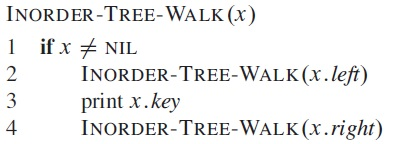The other two can be obtained by changing the position of the recursive calls. For general trees, only the last two orders are defined.

These orders can be followed manually by tracing the outline drawn around the tree:

• for preorder, visit node as you pass it going down,
• for inorder, visit node as you pass under it,
• for postorder, visit node as you pass it going up.
If the nodes in the tree are not sorted in any way, search can be done by tree walk, and takes Θ(n) time.

### 2. BST and search

To improve the efficiency of search, Binary Search Tree (BST) is built as a special binary tree with the following property: for every node in the tree, all nodes in its left subtree have smaller keys, and all nodes in its right subtree have larger keys. Example: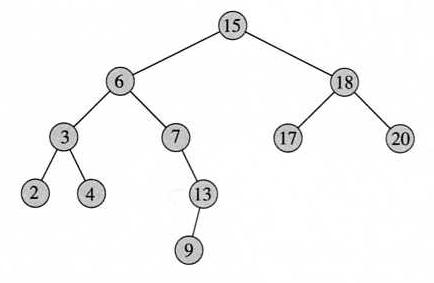Given this definition, an inorder walk of a binary search tree lists all of its node in order. In this sense, BST is "sorted" horizontally. In comparison, a heap is sorted vertically, so only maintains a partial order among all the nodes in the tree.

For such a binary tree, search is similar to binary search in an array.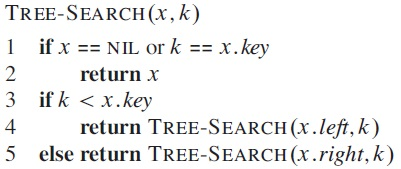The path the algorithm following is from the root to where a node is or should be in the tree, therefore the running time is proportional to the length of the path, and the worst case running time is proportional to the height of the tree.

The non-recursive version of the algorithm: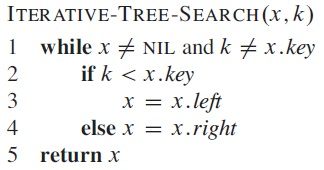We can take the search for minimum and maximum keys as special cases of the search operation. In these cases, the comparison in the path become unnecessary, and the algorithm simply goes to the end of one direction: left for the minimum and right for the maximum.

Given a node x in a binary search tree where all keys are distinct, the successor of the node is the node with the smallest key grater than x.key. In an inorder tree walk, this node will immediately follow x. The following algorithm assume the pointer to parent in each node. If x has a right subtree, then its successor is the minimum node in it, otherwise its successor is its closest ancestor that x is in its left subtree.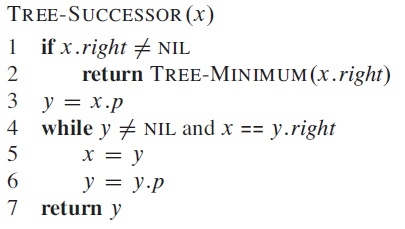The Tree-Predecessor algorithm is symmetric to this one.

Repeatedly calling Tree-Successor will give us a non-recursive inorder tree walk algorithm.

All the search algorithms on BST run in O(h) time, where h is the height of the tree.

### 3. Insertion and deletion in BST

When doing insertions and deletions in a binary search tree, the order among nodes must be maintained, so the result is still a binary search tree.

The following algorithm insert node z into BST T (assume z is not already in T):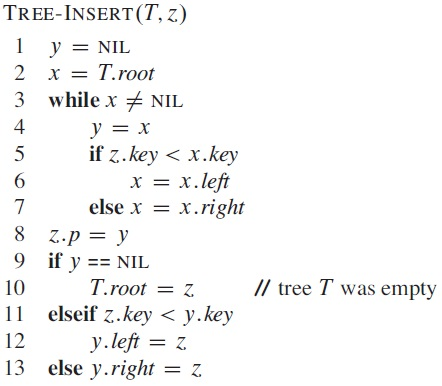In the algorithm, x traces a path to the insertion point, and y indicates the parent of x.

The deletion algorithm is more complicated, because after a non-leaf node is deleted, the "hole" in the structure needs to be filled by a leaf node. There are three possibilities:

1. To delete a leaf node (no children): disconnect it.
2. To delete a node with one child: bypass the node and directly connect to the child.
3. To delete a node with two children: find the smallest node in its right subtree (or the largest node in its left subtree), use it to replace the node to be deleted (which can be done by copying its info into the info field of the node to be deleted and then delete the minimum node instead). As that node can only have a right child, the situation becomes one of the above two.
This solution is realized with the help of an algorithm TRANSPLANT that replaces one subtree with root u as a child of its parent with another subtree with rootv.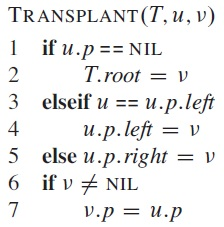In the following algorithm, z is an input argument referring to the node to be deleted from the BST T, and the local variable y refers to its successor.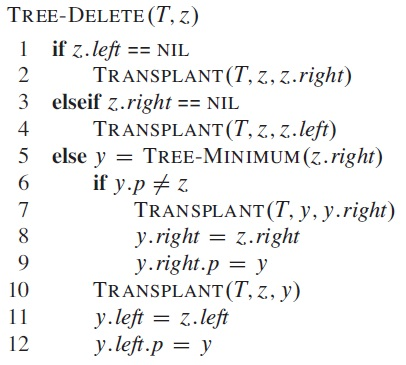Both above algorithms have run time O(h), where h is the height of the tree.

Since in BST all major operations have run time O(h), the height of a binary search tree determines the worst case run time. For a binary tree with n nodes, the best case (complete binary tree) is h = Θ(lg(n)), and the worst case (linear list) is h = Θ(n). A randomly formed BST has an expected height h = Θ(lg(n)).